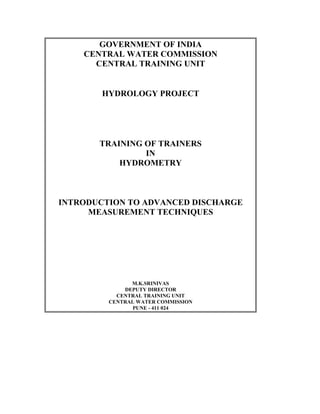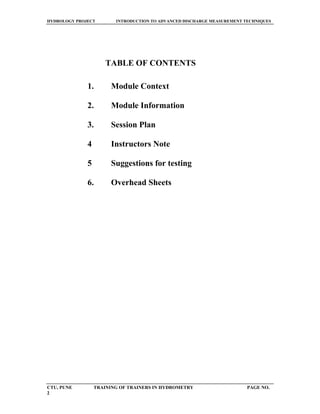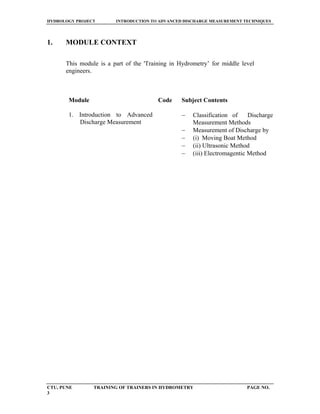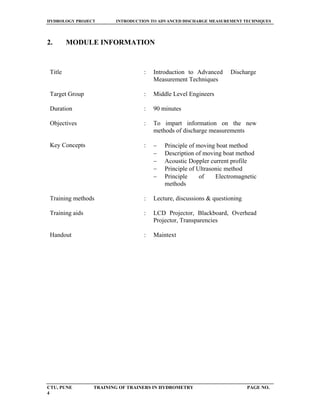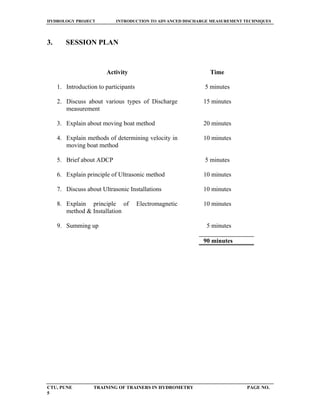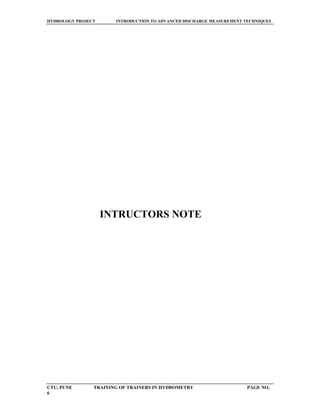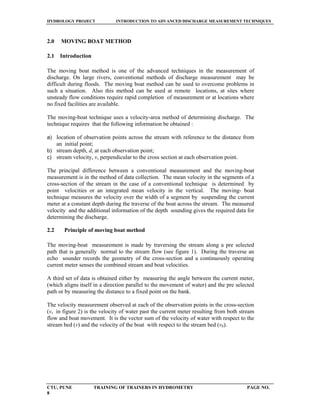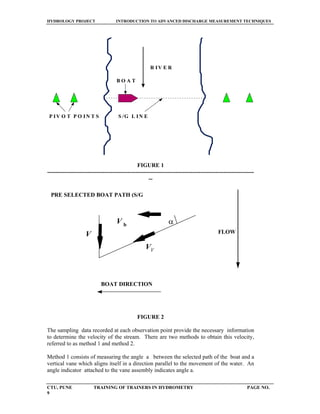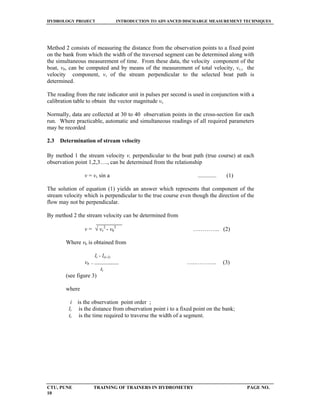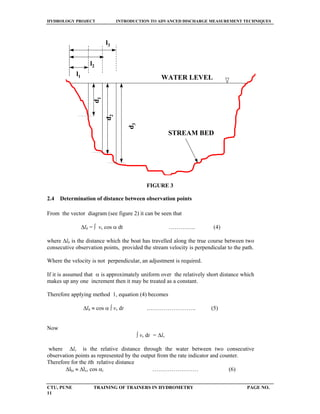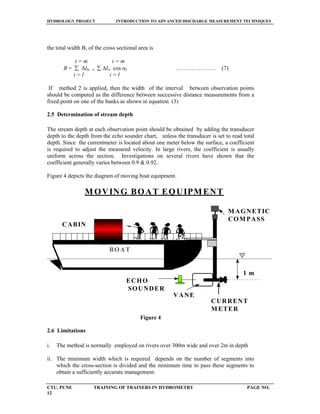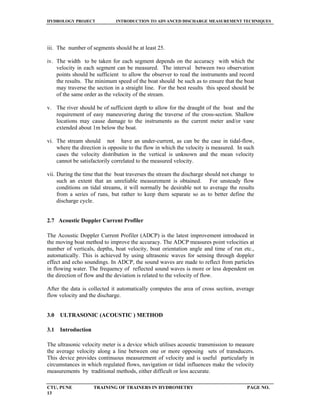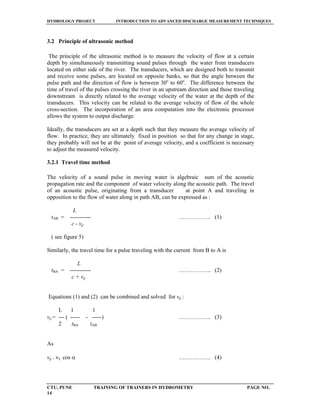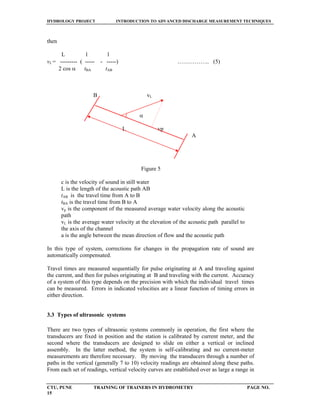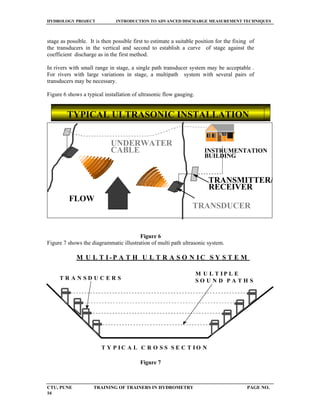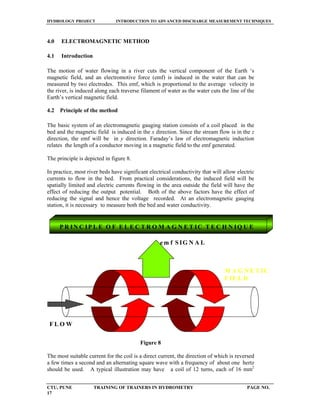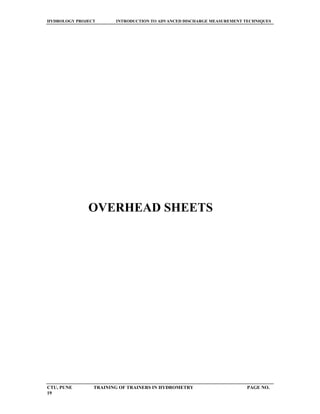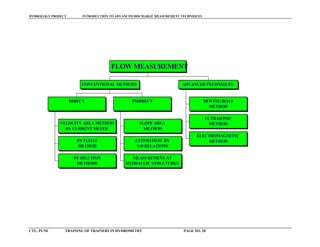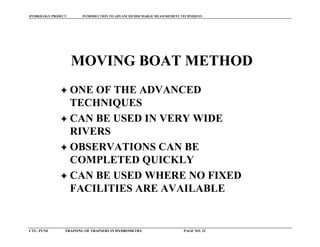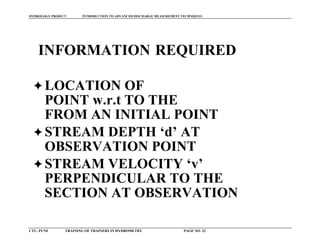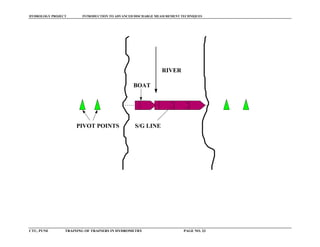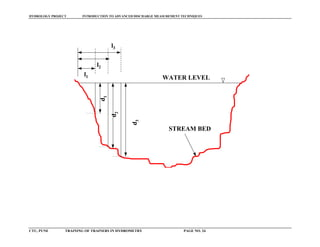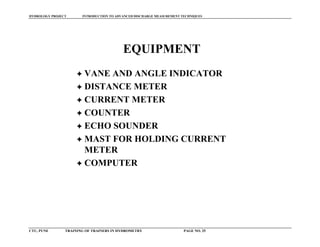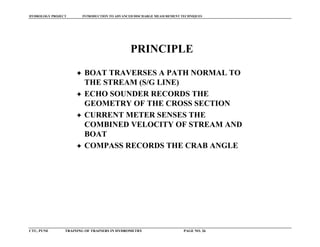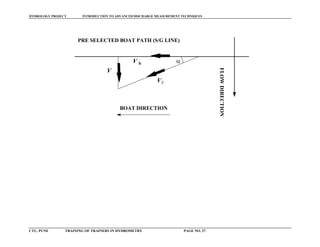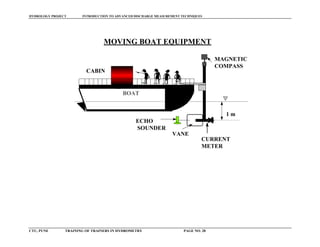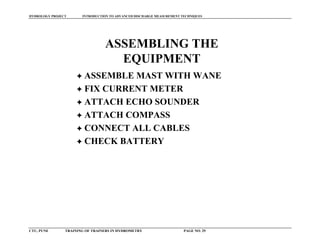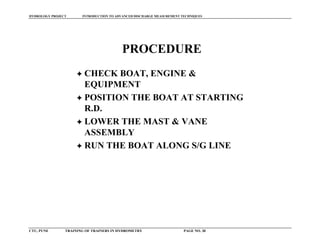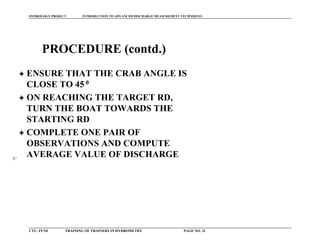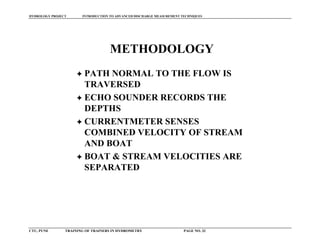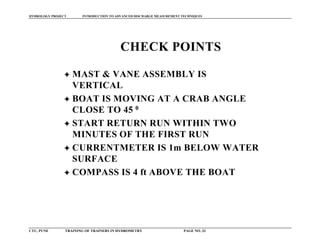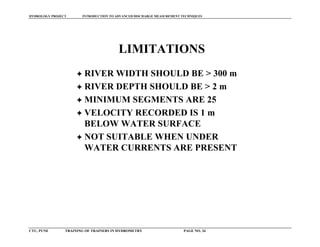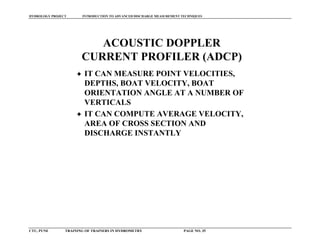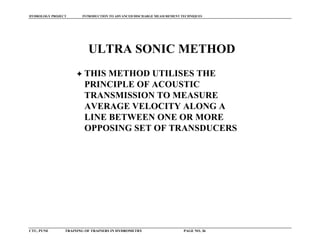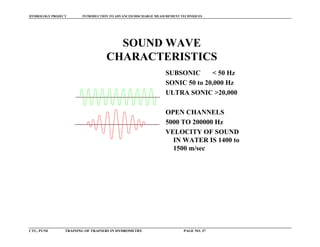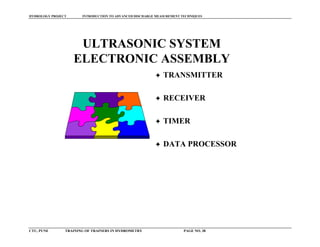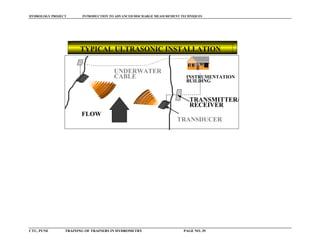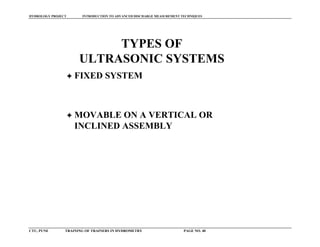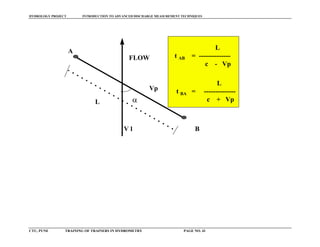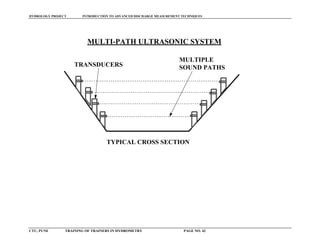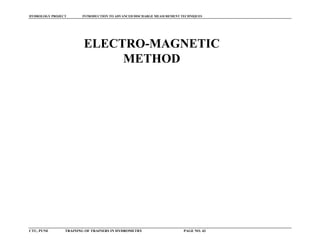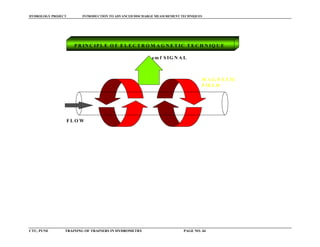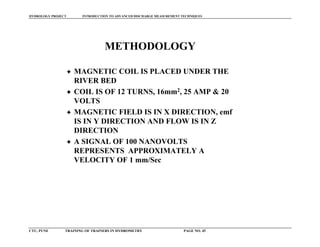Nächste SlideShare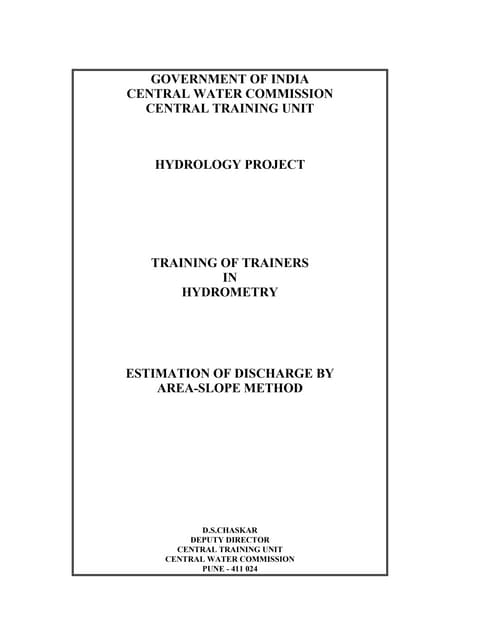Download-manuals-surface water-software-estimationofdischargebyarea-slopemethod
1 von 45Steram dicharge measurement Thim Mengly(ម៉េងលី,孟李)High Speed PIV ReportCharles FerrieraWater measurementHina Bhatu

1. GOVERNMENT OF INDIA CENTRAL WATER COMMISSION CENTRAL TRAINING UNIT HYDROLOGY PROJECT TRAINING OF TRAINERS IN HYDROMETRY INTRODUCTION TO ADVANCED DISCHARGE MEASUREMENT TECHNIQUES M.K.SRINIVAS DEPUTY DIRECTOR CENTRAL TRAINING UNIT CENTRAL WATER COMMISSION PUNE - 411 024
2. HYDROLOGY PROJECT INTRODUCTION TO ADVANCED DISCHARGE MEASUREMENT TECHNIQUES CTU, PUNE TRAINING OF TRAINERS IN HYDROMETRY PAGE NO. 2 TABLE OF CONTENTS 1. Module Context 2. Module Information 3. Session Plan 4 Instructors Note 5 Suggestions for testing 6. Overhead Sheets
3. HYDROLOGY PROJECT INTRODUCTION TO ADVANCED DISCHARGE MEASUREMENT TECHNIQUES CTU, PUNE TRAINING OF TRAINERS IN HYDROMETRY PAGE NO. 3 1. MODULE CONTEXT This module is a part of the 'Training in Hydrometry’ for middle level engineers. Module Code Subject Contents 1. Introduction to Advanced Discharge Measurement − Classification of Discharge Measurement Methods − Measurement of Discharge by − (i) Moving Boat Method − (ii) Ultrasonic Method − (iii) Electromagentic Method
4. HYDROLOGY PROJECT INTRODUCTION TO ADVANCED DISCHARGE MEASUREMENT TECHNIQUES CTU, PUNE TRAINING OF TRAINERS IN HYDROMETRY PAGE NO. 4 2. MODULE INFORMATION Title : Introduction to Advanced Discharge Measurement Techniques Target Group : Middle Level Engineers Duration : 90 minutes Objectives : To impart information on the new methods of discharge measurements Key Concepts : − Principle of moving boat method − Description of moving boat method − Acoustic Doppler current profile − Principle of Ultrasonic method − Principle of Electromagnetic methods Training methods : Lecture, discussions & questioning Training aids : LCD Projector, Blackboard, Overhead Projector, Transparencies Handout : Maintext
5. HYDROLOGY PROJECT INTRODUCTION TO ADVANCED DISCHARGE MEASUREMENT TECHNIQUES CTU, PUNE TRAINING OF TRAINERS IN HYDROMETRY PAGE NO. 5 3. SESSION PLAN Activity Time 1. Introduction to participants 5 minutes 2. Discuss about various types of Discharge measurement 15 minutes 3. Explain about moving boat method 20 minutes 4. Explain methods of determining velocity in moving boat method 10 minutes 5. Brief about ADCP 5 minutes 6. Explain principle of Ultrasonic method 10 minutes 7. Discuss about Ultrasonic Installations 10 minutes 8. Explain principle of Electromagnetic method & Installation 10 minutes 9. Summing up 5 minutes 90 minutes
6. HYDROLOGY PROJECT INTRODUCTION TO ADVANCED DISCHARGE MEASUREMENT TECHNIQUES CTU, PUNE TRAINING OF TRAINERS IN HYDROMETRY PAGE NO. 6 INTRUCTORS NOTE
7. HYDROLOGY PROJECT INTRODUCTION TO ADVANCED DISCHARGE MEASUREMENT TECHNIQUES CTU, PUNE TRAINING OF TRAINERS IN HYDROMETRY PAGE NO. 7 INTRODUCTION TO ADVANCED DISCHARGE MEASUREMENT TECHNIQUES 1.0 GENERAL Several methods are in vogue for determining discharge of a stream. The conventional methods are : Current meter method Dilution method Slope-Area method Measurements at Hydraulic structures Estimation by S-D Relationship Float method Three relatively new methods of flow measurement in open channels have gained importance in recent times. These are : Moving Boat method Ultrasonic method Electromagnetic method The discussion here is confined to the above three methods. The following chart shows the classification of various methods of discharge measurement. VELOCITY AREA METHODS BY CURRENT METER BY FLOAT METHOD BY DILUTION METHODS DIRECT SLOPE AREA METHOD ESTIMATION BY S-D RELATIONS MEASUREMENT AT HYDRAULIC STRUCTURES INDIRECT CONVENTIONAL METHODS MOVING BOAT METHOD ULTRASONIC (ACOUSTIC) METHOD ELECTROMAGNETIC METHOD ADVANCED TECHNIQUES FLOW MEASUREMENT
8. HYDROLOGY PROJECT INTRODUCTION TO ADVANCED DISCHARGE MEASUREMENT TECHNIQUES CTU, PUNE TRAINING OF TRAINERS IN HYDROMETRY PAGE NO. 8 2.0 MOVING BOAT METHOD 2.1 Introduction The moving boat method is one of the advanced techniques in the measurement of discharge. On large rivers, conventional methods of discharge measurement may be difficult during floods. The moving boat method can be used to overcome problems in such a situation. Also this method can be used at remote locations, at sites where unsteady flow conditions require rapid completion of measurement or at locations where no fixed facilities are available. The moving-boat technique uses a velocity-area method of determining discharge. The technique requires that the following information be obtained : a) location of observation points across the stream with reference to the distance from an initial point; b) stream depth, d, at each observation point; c) stream velocity, v, perpendicular to the cross section at each observation point. The principal difference between a conventional measurement and the moving-boat measurement is in the method of data collection. The mean velocity in the segments of a cross-section of the stream in the case of a conventional technique is determined by point velocities or an integrated mean velocity in the vertical. The moving- boat technique measures the velocity over the width of a segment by suspending the current meter at a constant depth during the traverse of the boat across the stream. The measured velocity and the additional information of the depth sounding gives the required data for determining the discharge. 2.2 Principle of moving boat method The moving-boat measurement is made by traversing the stream along a pre selected path that is generally normal to the stream flow (see figure 1). During the traverse an echo sounder records the geometry of the cross-section and a continuously operating current meter senses the combined stream and boat velocities. A third set of data is obtained either by measuring the angle between the current meter, (which aligns itself in a direction parallel to the movement of water) and the pre selected path or by measuring the distance to a fixed point on the bank. The velocity measurement observed at each of the observation points in the cross-section (vv in figure 2) is the velocity of water past the current meter resulting from both stream flow and boat movement. It is the vector sum of the velocity of water with respect to the stream bed (v) and the velocity of the boat with respect to the stream bed (vb).
9. HYDROLOGY PROJECT INTRODUCTION TO ADVANCED DISCHARGE MEASUREMENT TECHNIQUES CTU, PUNE TRAINING OF TRAINERS IN HYDROMETRY PAGE NO. 9 R IV E R S /G L IN EP IV O T P O IN T S B O A T FIGURE 1 ------------------------------------------------------------------------------------------------------------ -- α PRE SELECTED BOAT PATH (S/G FLOW V b V VV BOAT DIRECTION FIGURE 2 The sampling data recorded at each observation point provide the necessary information to determine the velocity of the stream. There are two methods to obtain this velocity, referred to as method 1 and method 2. Method 1 consists of measuring the angle a between the selected path of the boat and a vertical vane which aligns itself in a direction parallel to the movement of the water. An angle indicator attached to the vane assembly indicates angle a.
10. HYDROLOGY PROJECT INTRODUCTION TO ADVANCED DISCHARGE MEASUREMENT TECHNIQUES CTU, PUNE TRAINING OF TRAINERS IN HYDROMETRY PAGE NO. 10 Method 2 consists of measuring the distance from the observation points to a fixed point on the bank from which the width of the traversed segment can be determined along with the simultaneous measurement of time. From these data, the velocity component of the boat, vb, can be computed and by means of the measurement of total velocity, vv,, the velocity component, v, of the stream perpendicular to the selected boat path is determined. The reading from the rate indicator unit in pulses per second is used in conjunction with a calibration table to obtain the vector magnitude vv. Normally, data are collected at 30 to 40 observation points in the cross-section for each run. Where practicable, automatic and simultaneous readings of all required parameters may be recorded 2.3 Determination of stream velocity By method 1 the stream velocity v, perpendicular to the boat path (true course) at each observation point 1,2,3…., can be determined from the relationship v = vv sin a ............. (1) The solution of equation (1) yields an answer which represents that component of the stream velocity which is perpendicular to the true course even though the direction of the flow may not be perpendicular. By method 2 the stream velocity can be determined from _________ v = √ vv 2 - vb 2 ………….. (2) Where vb is obtained from li - l(i-1) vb = -------------------- …..……….. (3) ti (see figure 3) where i is the observation point order ; li is the distance from observation point i to a fixed point on the bank; ti is the time required to traverse the width of a segment.
11. HYDROLOGY PROJECT INTRODUCTION TO ADVANCED DISCHARGE MEASUREMENT TECHNIQUES CTU, PUNE TRAINING OF TRAINERS IN HYDROMETRY PAGE NO. 11 l1 l2 l3 d1 d2 d3 STREAM BED WATER LEVEL FIGURE 3 2.4 Determination of distance between observation points From the vector diagram (see figure 2) it can be seen that ∆lb = ∫ vv cos α dt ………….. (4) where ∆lb is the distance which the boat has travelled along the true course between two consecutive observation points, provided the stream velocity is perpendicular to the path. Where the velocity is not perpendicular, an adjustment is required. If it is assumed that α is approximately uniform over the relatively short distance which makes up any one increment then it may be treated as a constant. Therefore applying method 1, equation (4) becomes ∆lb ≈ cos α ∫ vv dt …………………….. (5) Now ∫ vv dt = ∆lv where ∆lv is the relative distance through the water between two consecutive observation points as represented by the output from the rate indicator and counter. Therefore for the ith relative distance ∆lbi ≈ ∆lvi cos αi …………………… (6)
12. HYDROLOGY PROJECT INTRODUCTION TO ADVANCED DISCHARGE MEASUREMENT TECHNIQUES CTU, PUNE TRAINING OF TRAINERS IN HYDROMETRY PAGE NO. 12 the total width B, of the cross sectional area is i = m i = m B = ∑ ∆lbi ≈ ∑ ∆lvi cos αi ………………… (7) i = l i = l If method 2 is applied, then the width of the interval between observation points should be computed as the difference between successive distance measurements from a fixed point on one of the banks as shown in equation (3) 2.5 Determination of stream depth The stream depth at each observation point should be obtained by adding the transducer depth to the depth from the echo sounder chart, unless the transducer is set to read total depth. Since the currentmeter is located about one meter below the surface, a coefficient is required to adjust the measured velocity. In large rivers, the coefficient is usually uniform across the section. Investigations on several rivers have shown that the coefficient generally varies between 0.9 & 0.92. Figure 4 depicts the diagram of moving boat equipment. 1 m MAGNETIC COMPASS ECHO SOUNDER CURRENT METER VANE CABIN M OVING BOAT EQUIPMENT BOAT Figure 4 2.6 Limitations i. The method is normally employed on rivers over 300m wide and over 2m in depth ii. The minimum width which is required depends on the number of segments into which the cross-section is divided and the minimum time to pass these segments to obtain a sufficiently accurate management.
13. HYDROLOGY PROJECT INTRODUCTION TO ADVANCED DISCHARGE MEASUREMENT TECHNIQUES CTU, PUNE TRAINING OF TRAINERS IN HYDROMETRY PAGE NO. 13 iii. The number of segments should be at least 25. iv. The width to be taken for each segment depends on the accuracy with which the velocity in each segment can be measured. The interval between two observation points should be sufficient to allow the observer to read the instruments and record the results. The minimum speed of the boat should be such as to ensure that the boat may traverse the section in a straight line. For the best results this speed should be of the same order as the velocity of the stream. v. The river should be of sufficient depth to allow for the draught of the boat and the requirement of easy maneuvering during the traverse of the cross-section. Shallow locations may cause damage to the instruments as the current meter and/or vane extended about 1m below the boat. vi. The stream should not have an under-current, as can be the case in tidal-flow, where the direction is opposite to the flow in which the velocity is measured. In such cases the velocity distribution in the vertical is unknown and the mean velocity cannot be satisfactorily correlated to the measured velocity. vii. During the time that the boat traverses the stream the discharge should not change to such an extent that an unreliable measurement is obtained. For unsteady flow conditions on tidal streams, it will normally be desirable not to average the results from a series of runs, but rather to keep them separate so as to better define the discharge cycle. 2.7 Acoustic Doppler Current Profiler The Acoustic Doppler Current Profiler (ADCP) is the latest improvement introduced in the moving boat method to improve the accuracy. The ADCP measures point velocities at number of verticals, depths, boat velocity, boat orientation angle and time of run etc., automatically. This is achieved by using ultrasonic waves for sensing through doppler effect and echo soundings. In ADCP, the sound waves are made to reflect from particles in flowing water. The frequency of reflected sound waves is more or less dependent on the direction of flow and the deviation is related to the velocity of flow. After the data is collected it automatically computes the area of cross section, average flow velocity and the discharge. 3.0 ULTRASONIC (ACOUSTIC ) METHOD 3.1 Introduction The ultrasonic velocity meter is a device which utilises acoustic transmission to measure the average velocity along a line between one or more opposing sets of transducers. This device provides continuous measurement of velocity and is useful particularly in circumstances in which regulated flows, navigation or tidal influences make the velocity measurements by traditional methods, either difficult or less accurate.
14. HYDROLOGY PROJECT INTRODUCTION TO ADVANCED DISCHARGE MEASUREMENT TECHNIQUES CTU, PUNE TRAINING OF TRAINERS IN HYDROMETRY PAGE NO. 14 3.2 Principle of ultrasonic method The principle of the ultrasonic method is to measure the velocity of flow at a certain depth by simultaneously transmitting sound pulses through the water from transducers located on either side of the river. The transducers, which are designed both to transmit and receive some pulses, are located on opposite banks, so that the angle between the pulse path and the direction of flow is between 30o to 60o . The difference between the time of travel of the pulses crossing the river in an upstream direction and those traveling downstream is directly related to the average velocity of the water at the depth of the transducers. This velocity can be related to the average velocity of flow of the whole cross-section. The incorporation of an area computation into the electronic processor allows the system to output discharge. Ideally, the transducers are set at a depth such that they measure the average velocity of flow. In practice, they are ultimately fixed in position so that for any change in stage, they probably will not be at the point of average velocity, and a coefficient is necessary to adjust the measured velocity. 3.2.1 Travel time method The velocity of a sound pulse in moving water is algebraic sum of the acoustic propagation rate and the component of water velocity along the acoustic path. The travel of an acoustic pulse, originating from a transducer at point A and traveling in opposition to the flow of water along in path AB, can be expressed as : L tAB = ----------- …………….. (1) c - vp ( see figure 5) Similarly, the travel time for a pulse traveling with the current from B to A is L tBA = ----------- …………….. (2) c + vp Equations (1) and (2) can be combined and solved for vp : L 1 1 vp = --- ( ----- - -----) …………….. (3) 2 tBA tAB As vp = vL cos α …………….. (4)
15. HYDROLOGY PROJECT INTRODUCTION TO ADVANCED DISCHARGE MEASUREMENT TECHNIQUES CTU, PUNE TRAINING OF TRAINERS IN HYDROMETRY PAGE NO. 15 then L 1 1 vL = --------- ( ----- - -----) …………….. (5) 2 cos α tBA tAB B vL α L vp A Figure 5 c is the velocity of sound in still water L is the length of the acoustic path AB tAB is the travel time from A to B tBA is the travel time from B to A vp is the component of the measured average water velocity along the acoustic path vL is the average water velocity at the elevation of the acoustic path parallel to the axis of the channel a is the angle between the mean direction of flow and the acoustic path In this type of system, corrections for changes in the propagation rate of sound are automatically compensated. Travel times are measured sequentially for pulse originating at A and traveling against the current, and then for pulses originating at B and traveling with the current. Accuracy of a system of this type depends on the precision with which the individual travel times can be measured. Errors in indicated velocities are a linear function of timing errors in either direction. 3.3 Types of ultrasonic systems There are two types of ultrasonic systems commonly in operation, the first where the transducers are fixed in position and the station is calibrated by current meter, and the second where the transducers are designed to slide on either a vertical or inclined assembly. In the latter method, the system is self-calibrating and no current-meter measurements are therefore necessary. By moving the transducers through a number of paths in the vertical (generally 7 to 10) velocity readings are obtained along these paths. From each set of readings, vertical velocity curves are established over as large a range in
16. HYDROLOGY PROJECT INTRODUCTION TO ADVANCED DISCHARGE MEASUREMENT TECHNIQUES CTU, PUNE TRAINING OF TRAINERS IN HYDROMETRY PAGE NO. 16 stage as possible. It is then possible first to estimate a suitable position for the fixing of the transducers in the vertical and second to establish a curve of stage against the coefficient discharge as in the first method. In rivers with small range in stage, a single path transducer system may be acceptable . For rivers with large variations in stage, a multipath system with several pairs of transducers may be necessary. Figure 6 shows a typical installation of ultrasonic flow gauging. FLOW TRANSDUCER TRANSMITTER/ RECEIVER INSTRUMENTATION BUILDING UNDERWATER CABLE TYPICAL ULTRASONIC INSTALLATION Figure 6 Figure 7 shows the diagrammatic illustration of multi path ultrasonic system. M U L T I P L E S O U N D P A T H ST R A N S D U C E R S M U L T I -P A T H U L T R A S O N I C S Y S T E M T Y P I C A L C R O S S S E C T I O N Figure 7
17. HYDROLOGY PROJECT INTRODUCTION TO ADVANCED DISCHARGE MEASUREMENT TECHNIQUES CTU, PUNE TRAINING OF TRAINERS IN HYDROMETRY PAGE NO. 17 4.0 ELECTROMAGNETIC METHOD 4.1 Introduction The motion of water flowing in a river cuts the vertical component of the Earth ‘s magnetic field, and an electromotive force (emf) is induced in the water that can be measured by two electrodes. This emf, which is proportional to the average velocity in the river, is induced along each traverse filament of water as the water cuts the line of the Earth’s vertical magnetic field. 4.2 Principle of the method The basic system of an electromagnetic gauging station consists of a coil placed in the bed and the magnetic field is induced in the x direction. Since the stream flow is in the z direction, the emf will be in y direction. Faraday’s law of electromagnetic induction relates the length of a conductor moving in a magnetic field to the emf generated. The principle is depicted in figure 8. In practice, most river beds have significant electrical conductivity that will allow electric currents to flow in the bed. From practical considerations, the induced field will be spatially limited and electric currents flowing in the area outside the field will have the effect of reducing the output potential. Both of the above factors have the effect of reducing the signal and hence the voltage recorded. At an electromagnetic gauging station, it is necessary to measure both the bed and water conductivity. F L O W M A G N E T IC F IE L D em f S IG N A L P R IN C IP L E O F E L E C T R O M A G N E T IC T E C H N IQ U E Figure 8 The most suitable current for the coil is a direct current, the direction of which is reversed a few times a second and an alternating square wave with a frequency of about one hertz should be used. A typical illustration may have a coil of 12 turns, each of 16 mm2
18. HYDROLOGY PROJECT INTRODUCTION TO ADVANCED DISCHARGE MEASUREMENT TECHNIQUES CTU, PUNE TRAINING OF TRAINERS IN HYDROMETRY PAGE NO. 18 double PVC insulated cable, and supplied with 25 ampere with a voltage across the coil of about 20 volts. The electromagnetic method will be suitable for use in rivers with weed growth, high sediment concentration, or unstable bed conditions and gives a continuous record of the average velocity in the cross-section that can be combined with stage to given an on-site output of discharge. 4.3 Accuracy The accuracy depends on the signal processing equipment detecting and measuring small potentials sensed at the voltage probes. It is possible to detect a signal of 100 nano volts (10-9 volts), which represents a velocity of approximately 1 mm s-1 . The electromagnetic gauging station requires on-site calibration by current meter or other means and a relation established between discharge and output. REFERENCES 1) International organisation for standardisation, 1979: ‘ Measurement of liquid flow in open channels - Moving boat method’ ISO 4369, Geneva. 2) International organisation for standardisation, 1985: ‘ Liquid flow measurement in open channels - Ultrasonic ( Acoustic) velocity meters’ ISO 6418, Geneva. 3) World Meteorological Organisation, 1994: ‘Guide to Hydrological Practices - Data acquisition & processing, analysis, forecasting & other applications.’ WMO report No.168., Geneva.
19. HYDROLOGY PROJECT INTRODUCTION TO ADVANCED DISCHARGE MEASUREMENT TECHNIQUES CTU, PUNE TRAINING OF TRAINERS IN HYDROMETRY PAGE NO. 19 OVERHEAD SHEETS
20. HYDROLOGY PROJECT INTRODUCTION TO ADVANCED DISCHARGE MEASUREMENT TECHNIQUES CTU, PUNE TRAINING OF TRAINERS IN HYDROMETRY PAGE NO. 20 VELOCITY AREA METHOD BY CURRENT METER BY FLOAT METHOD BY DILUTION METHODS DIRECT SLOPE AREA METHOD ESTIMATION BY S-D RELATIONS MEASUREMENT AT HYDRAULIC STRUCTURES INDIRECT CONVENTIONAL METHODS MOVING BOAT METHOD ULTRASONIC METHOD ELECTROMAGNETIC METHOD ADVANCED TECHNIQUES FLOW MEASUREMENT
21. HYDROLOGY PROJECT INTRODUCTION TO ADVANCED DISCHARGE MEASUREMENT TECHNIQUES CTU, PUNE TRAINING OF TRAINERS IN HYDROMETRY PAGE NO. 21 MOVING BOAT METHOD ONE OF THE ADVANCED TECHNIQUES CAN BE USED IN VERY WIDE RIVERS OBSERVATIONS CAN BE COMPLETED QUICKLY CAN BE USED WHERE NO FIXED FACILITIES ARE AVAILABLE
22. HYDROLOGY PROJECT INTRODUCTION TO ADVANCED DISCHARGE MEASUREMENT TECHNIQUES CTU, PUNE TRAINING OF TRAINERS IN HYDROMETRY PAGE NO. 22 INFORMATION REQUIRED LOCATION OF POINT w.r.t TO THE FROM AN INITIAL POINT STREAM DEPTH ‘d’ AT OBSERVATION POINT STREAM VELOCITY ‘v’ PERPENDICULAR TO THE SECTION AT OBSERVATION
23. HYDROLOGY PROJECT INTRODUCTION TO ADVANCED DISCHARGE MEASUREMENT TECHNIQUES CTU, PUNE TRAINING OF TRAINERS IN HYDROMETRY PAGE NO. 23 RIVER S/G LINEPIVOT POINTS BOAT
24. HYDROLOGY PROJECT INTRODUCTION TO ADVANCED DISCHARGE MEASUREMENT TECHNIQUES CTU, PUNE TRAINING OF TRAINERS IN HYDROMETRY PAGE NO. 24 l1 l2 l3 d1 d2 d3 STREAM BED WATER LEVEL
25. HYDROLOGY PROJECT INTRODUCTION TO ADVANCED DISCHARGE MEASUREMENT TECHNIQUES CTU, PUNE TRAINING OF TRAINERS IN HYDROMETRY PAGE NO. 25 EQUIPMENT VANE AND ANGLE INDICATOR DISTANCE METER CURRENT METER COUNTER ECHO SOUNDER MAST FOR HOLDING CURRENT METER COMPUTER
26. HYDROLOGY PROJECT INTRODUCTION TO ADVANCED DISCHARGE MEASUREMENT TECHNIQUES CTU, PUNE TRAINING OF TRAINERS IN HYDROMETRY PAGE NO. 26 PRINCIPLE BOAT TRAVERSES A PATH NORMAL TO THE STREAM (S/G LINE) ECHO SOUNDER RECORDS THE GEOMETRY OF THE CROSS SECTION CURRENT METER SENSES THE COMBINED VELOCITY OF STREAM AND BOAT COMPASS RECORDS THE CRAB ANGLE
27. HYDROLOGY PROJECT INTRODUCTION TO ADVANCED DISCHARGE MEASUREMENT TECHNIQUES CTU, PUNE TRAINING OF TRAINERS IN HYDROMETRY PAGE NO. 27 α PRE SELECTED BOAT PATH (S/G LINE) FLOWDIRECTION V b V VV BOAT DIRECTION
28. HYDROLOGY PROJECT INTRODUCTION TO ADVANCED DISCHARGE MEASUREMENT TECHNIQUES CTU, PUNE TRAINING OF TRAINERS IN HYDROMETRY PAGE NO. 28 1 m MAGNETIC COMPASS ECHO SOUNDER CURRENT METER VANE CABIN MOVING BOAT EQUIPMENT BOAT
29. HYDROLOGY PROJECT INTRODUCTION TO ADVANCED DISCHARGE MEASUREMENT TECHNIQUES CTU, PUNE TRAINING OF TRAINERS IN HYDROMETRY PAGE NO. 29 ASSEMBLING THE EQUIPMENT ASSEMBLE MAST WITH WANE FIX CURRENT METER ATTACH ECHO SOUNDER ATTACH COMPASS CONNECT ALL CABLES CHECK BATTERY
30. HYDROLOGY PROJECT INTRODUCTION TO ADVANCED DISCHARGE MEASUREMENT TECHNIQUES CTU, PUNE TRAINING OF TRAINERS IN HYDROMETRY PAGE NO. 30 PROCEDURE CHECK BOAT, ENGINE & EQUIPMENT POSITION THE BOAT AT STARTING R.D. LOWER THE MAST & VANE ASSEMBLY RUN THE BOAT ALONG S/G LINE
31. HYDROLOGY PROJECT INTRODUCTION TO ADVANCED DISCHARGE MEASUREMENT TECHNIQUES CTU, PUNE TRAINING OF TRAINERS IN HYDROMETRY PAGE NO. 31 'C' PROCEDURE (contd.) ENSURE THAT THE CRAB ANGLE IS CLOSE TO 45 0 ON REACHING THE TARGET RD, TURN THE BOAT TOWARDS THE STARTING RD COMPLETE ONE PAIR OF OBSERVATIONS AND COMPUTE AVERAGE VALUE OF DISCHARGE
32. HYDROLOGY PROJECT INTRODUCTION TO ADVANCED DISCHARGE MEASUREMENT TECHNIQUES CTU, PUNE TRAINING OF TRAINERS IN HYDROMETRY PAGE NO. 32 METHODOLOGY PATH NORMAL TO THE FLOW IS TRAVERSED ECHO SOUNDER RECORDS THE DEPTHS CURRENTMETER SENSES COMBINED VELOCITY OF STREAM AND BOAT BOAT & STREAM VELOCITIES ARE SEPARATED
33. HYDROLOGY PROJECT INTRODUCTION TO ADVANCED DISCHARGE MEASUREMENT TECHNIQUES CTU, PUNE TRAINING OF TRAINERS IN HYDROMETRY PAGE NO. 33 CHECK POINTS MAST & VANE ASSEMBLY IS VERTICAL BOAT IS MOVING AT A CRAB ANGLE CLOSE TO 45 0 START RETURN RUN WITHIN TWO MINUTES OF THE FIRST RUN CURRENTMETER IS 1m BELOW WATER SURFACE COMPASS IS 4 ft ABOVE THE BOAT
34. HYDROLOGY PROJECT INTRODUCTION TO ADVANCED DISCHARGE MEASUREMENT TECHNIQUES CTU, PUNE TRAINING OF TRAINERS IN HYDROMETRY PAGE NO. 34 LIMITATIONS RIVER WIDTH SHOULD BE > 300 m RIVER DEPTH SHOULD BE > 2 m MINIMUM SEGMENTS ARE 25 VELOCITY RECORDED IS 1 m BELOW WATER SURFACE NOT SUITABLE WHEN UNDER WATER CURRENTS ARE PRESENT
35. HYDROLOGY PROJECT INTRODUCTION TO ADVANCED DISCHARGE MEASUREMENT TECHNIQUES CTU, PUNE TRAINING OF TRAINERS IN HYDROMETRY PAGE NO. 35 ACOUSTIC DOPPLER CURRENT PROFILER (ADCP) IT CAN MEASURE POINT VELOCITIES, DEPTHS, BOAT VELOCITY, BOAT ORIENTATION ANGLE AT A NUMBER OF VERTICALS IT CAN COMPUTE AVERAGE VELOCITY, AREA OF CROSS SECTION AND DISCHARGE INSTANTLY
36. HYDROLOGY PROJECT INTRODUCTION TO ADVANCED DISCHARGE MEASUREMENT TECHNIQUES CTU, PUNE TRAINING OF TRAINERS IN HYDROMETRY PAGE NO. 36 ULTRA SONIC METHOD THIS METHOD UTILISES THE PRINCIPLE OF ACOUSTIC TRANSMISSION TO MEASURE AVERAGE VELOCITY ALONG A LINE BETWEEN ONE OR MORE OPPOSING SET OF TRANSDUCERS
37. HYDROLOGY PROJECT INTRODUCTION TO ADVANCED DISCHARGE MEASUREMENT TECHNIQUES CTU, PUNE TRAINING OF TRAINERS IN HYDROMETRY PAGE NO. 37 SOUND WAVE CHARACTERISTICS SUBSONIC < 50 Hz SONIC 50 to 20,000 Hz ULTRA SONIC >20,000 OPEN CHANNELS 5000 TO 200000 Hz VELOCITY OF SOUND IN WATER IS 1400 to 1500 m/sec
38. HYDROLOGY PROJECT INTRODUCTION TO ADVANCED DISCHARGE MEASUREMENT TECHNIQUES CTU, PUNE TRAINING OF TRAINERS IN HYDROMETRY PAGE NO. 38 ULTRASONIC SYSTEM ELECTRONIC ASSEMBLY TRANSMITTER RECEIVER TIMER DATA PROCESSOR
39. HYDROLOGY PROJECT INTRODUCTION TO ADVANCED DISCHARGE MEASUREMENT TECHNIQUES CTU, PUNE TRAINING OF TRAINERS IN HYDROMETRY PAGE NO. 39 FLOW TRANSDUCER TRANSMITTER/ RECEIVER INSTRUMENTATION BUILDING UNDERWATER CABLE TYPICAL ULTRASONIC INSTALLATION
40. HYDROLOGY PROJECT INTRODUCTION TO ADVANCED DISCHARGE MEASUREMENT TECHNIQUES CTU, PUNE TRAINING OF TRAINERS IN HYDROMETRY PAGE NO. 40 TYPES OF ULTRASONIC SYSTEMS FIXED SYSTEM MOVABLE ON A VERTICAL OR INCLINED ASSEMBLY
41. HYDROLOGY PROJECT INTRODUCTION TO ADVANCED DISCHARGE MEASUREMENT TECHNIQUES CTU, PUNE TRAINING OF TRAINERS IN HYDROMETRY PAGE NO. 41 L B A FLOW α Vp V l L t AB = -------------- c - Vp L t BA = -------------- c + Vp
42. HYDROLOGY PROJECT INTRODUCTION TO ADVANCED DISCHARGE MEASUREMENT TECHNIQUES CTU, PUNE TRAINING OF TRAINERS IN HYDROMETRY PAGE NO. 42 MULTIPLE SOUND PATHSTRANSDUCERS MULTI-PATH ULTRASONIC SYSTEM TYPICAL CROSS SECTION
43. HYDROLOGY PROJECT INTRODUCTION TO ADVANCED DISCHARGE MEASUREMENT TECHNIQUES CTU, PUNE TRAINING OF TRAINERS IN HYDROMETRY PAGE NO. 43 ELECTRO-MAGNETIC METHOD
44. HYDROLOGY PROJECT INTRODUCTION TO ADVANCED DISCHARGE MEASUREMENT TECHNIQUES CTU, PUNE TRAINING OF TRAINERS IN HYDROMETRY PAGE NO. 44 F L O W M A G N E T IC F IE L D em f S IG N A L P R IN C IP L E O F E L E C T R O M A G N E T IC T E C H N IQ U E
45. HYDROLOGY PROJECT INTRODUCTION TO ADVANCED DISCHARGE MEASUREMENT TECHNIQUES CTU, PUNE TRAINING OF TRAINERS IN HYDROMETRY PAGE NO. 45 METHODOLOGY MAGNETIC COIL IS PLACED UNDER THE RIVER BED COIL IS OF 12 TURNS, 16mm2, 25 AMP & 20 VOLTS MAGNETIC FIELD IS IN X DIRECTION, emf IS IN Y DIRECTION AND FLOW IS IN Z DIRECTION A SIGNAL OF 100 NANOVOLTS REPRESENTS APPROXIMATELY A VELOCITY OF 1 mm/Sec